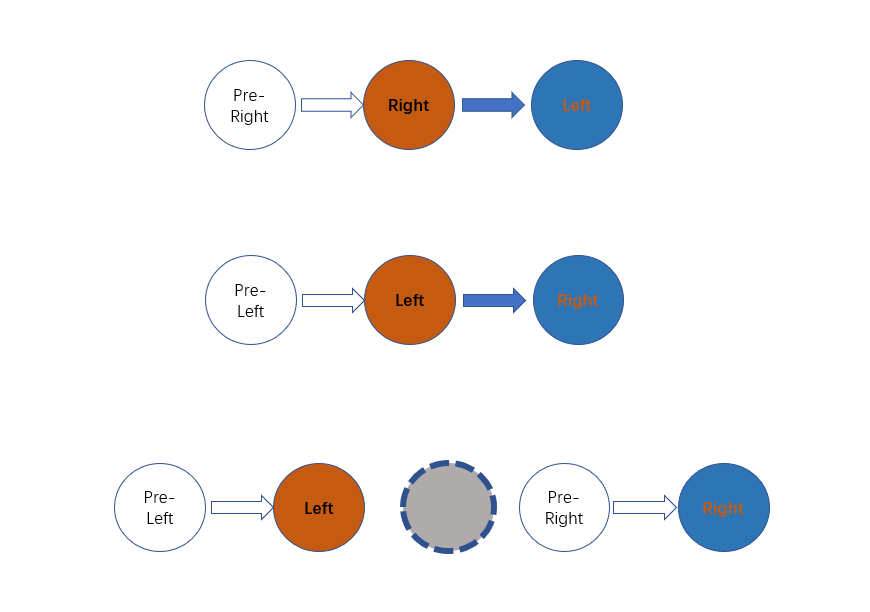By Long Luo

Here shows 3 Approaches to slove this problem: ArrayList and Two Pointers.

# Intuition

Since the $\texttt{ListNode}$ only contain values, we can just just swap the values of two nodes. It’s very easy. All we need to do is to find these two nodes and swap their values.

If we swap nodes, it will be more difficult than swap values.

# ArrayList(Swapping Values)

We can use an $\texttt{ArrayList}$ to record all the nodes of the linked list. We can just swap the values of two nodes.

## Analysis

• Time Complexity: $O(n)$
• Space Complexity: $O(n)$

# Two Pointers(Swapping Values)

The space complexity of Method $1$ is $O(n)$. We can use Two Pointers to make it to $O(1)$.

1. Find the $k-th$ node from the front which is left.
2. Make left node as the current node, right node from the front, when the current node reach end, right node is just the $k-th$ last element.
3. Swap their values.

## Analysis

• Time Complexity: $O(n)$
• Space Complexity: $O(1)$

# Two Pointers(Swapping Nodes)

In fact, Swapping Nodes will be more difficult. There are some corner cases.

We need to store both the previous and current nodes of these two nodes, denoted them as $\textit{preLeft}$ and $\textit{preRight}$.

However, here are 3 corner cases, as the bellow image shows.We must handle these 3 cases.

## Analysis

• Time Complexity: $O(n)$
• Space Complexity: $O(1)$

All suggestions are welcome.
If you have any query or suggestion please comment below.
Please upvote👍 if you like💗 it. Thank you:-)

Explore More Leetcode Solutions. 😉😃💗